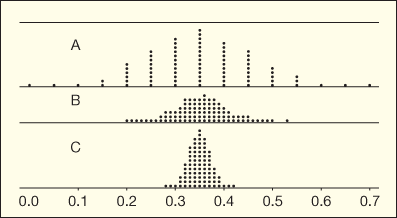Question
6 views
Proportion of US Residents Less than 25 Years Old
The US Census indicates that 35% of US residents are less than 25 years old. Figure 3.7 shows possible sampling distributions for the proportion of a sample less than 25 years old, for samples of size n = 20, n = 100, and n = 500.
Figure 3.7   Match the dotplots with the sample size
(a)
Which distribution goes with which sample size?
b)
If we use a proportion p^, based on a sample of size n = 20, to estimate the population parameter p = 0.35, would it be very surprising to get an estimate that is off by more than 0.10 (that is, the sample proportion is less than 0.25 or greater than 0.45)? How about with a sample of size n = 100? How about with a sample of size n = 500?
(c)
Repeat part (b) if we ask about the sample proportion being off by just 0.05 or more.
(d)
Using parts (b) and (c), comment on the effect that sample size has on the accuracy of an estimate.help_outlineImage TranscriptioncloseП 0.0 0.1 0.2 0.3 0.4 0.5 0.6 0.7 fullscreen
check_circle

Step 1

(a)

The important thing is that, as the sample size increases the accuracy increases and the spread of the data decreases.

A sample size n=20 goes with the distribution A.

A sample size n=100 goes with the distribution B.

A sample size n=500 goes with the distribution C.

Step 2

(b)

No, because from the dot plot A, it can be observed that there are points that are less than 0.25 or greater than 0.45. Thus, it would not be surprising to get an estimate that is off by more than 0.10.

No, for a sample size n=100, it is not surprising to get an estimate that is off by more than 0.10 because there are few points that lies close to 0.25 and above 0.45.

Yes, for a sample size n=500, it is surprising to get an estimate that is off by more than 0.10 because all the points lies between 0.30 and 0.40.

Step 3

(c)

No, because from the dot plot A it can be observed that there are many points that are less than 0.30 or greater than 0.40. Thus, it would not be surprising to get an estimate that is off by more than 0.10.

No, for a sample size n=100, it is not that surprising to get an estimate that is off by more tha...

### Want to see the full answer?

See Solution

#### Want to see this answer and more?

Solutions are written by subject experts who are available 24/7. Questions are typically answered within 1 hour.*

See Solution
*Response times may vary by subject and question.
Tagged in